# Algebra II : Multiplying and Dividing Logarithms

## Example Questions

### Example Question #1 : Multiplying And Dividing Logarithms

Rewrite the following logarithmic expression in expanded form (i.e. as a sum and/or difference):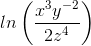Possible Answers: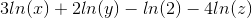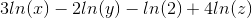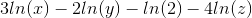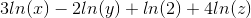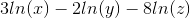Correct answer:Explanation:

By logarithmic properties: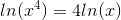;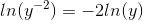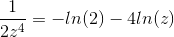Combining these three terms gives the correct answer:### Example Question #1 : Simplifying Logarithms

Which of the following is equivalent to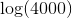Possible Answers: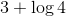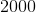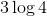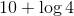Correct answer:Explanation:

Recall that log implies baseif not indicated.Then, we break up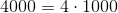. Thus, we have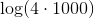.

Our log rules indicate that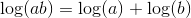.

So we are really interested in,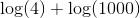.

Since we are interested in log base, we can solve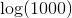without a calculator.

We know that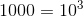, and thus by the definition of log we have that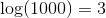.

Therefore, we have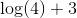### Example Question #3 : Multiplying And Dividing Logarithms

Find the value of the Logarithmic Expression.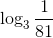Possible Answers: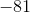Correct answer:Explanation:

Use the change of base formula to solve this equation.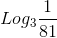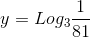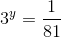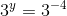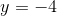### Example Question #4 : Multiplying And Dividing Logarithms

What is another way of expressing the following?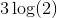Possible Answers: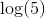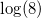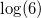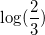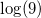Correct answer:Explanation:

Use the rule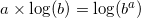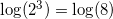### Example Question #5 : Multiplying And Dividing Logarithms

Expand this logarithm: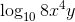Possible Answers: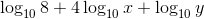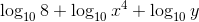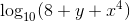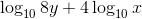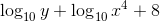Correct answer:Explanation:

In order to solve this problem you must understand the product property of logarithms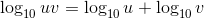and the power property of logarithms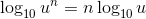. Note that these apply to logs of all bases not just base 10.log of multiple terms is the log of each individual one:now use the power property to move the exponent over:### Example Question #6 : Multiplying And Dividing Logarithms

Which of the following is equivalent to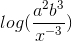?

Possible Answers: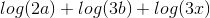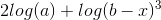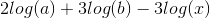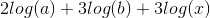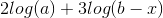Correct answer:Explanation:

We can rewrite the terms of the inner quantity.  Change the negative exponent into a fraction.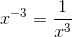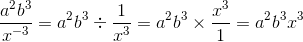This means that: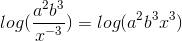Split up these logarithms by addition.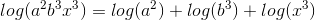According to the log rules, the powers can be transferred in front of the logs as coefficients.

The answer is:### Example Question #7 : Multiplying And Dividing Logarithms

Many textbooks use the following convention for logarithms: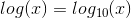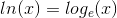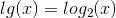Solve: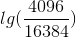Possible Answers:Correct answer:Explanation:

Remembering the rules for logarithms, we know that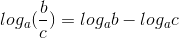.

This tells us that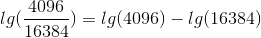.

This becomes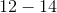, which is.

### All Algebra II Resources